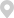• 上市培训
• 市场培育
• 市场培育合作
• 市场培育基地
• 金融办之家
• 问题解答
• 发行上市摘要
• 发行上市条件
• 聘请中介机构
• 企业规范运作
• 发行审核流程
• 承销与发行
• 股票发行审核关注要点
• 特殊行业改制上市
• 信息披露
• 信息披露总览
• 交易备忘
• 本所公告
• 通知公告
• 停复牌
• 上市公司
• 固收产品
• 基金
• 交易信息
• 交易公开信息
• 大宗交易信息
• 权益类证券大宗交易
• 债券大宗交易
• 债券大宗交易（实时）
• 权证持有人信息
• QFII/RQFII/深股通投资者信息
• 要约收购
• 非流通股转让
• 优先股信息
• 融资融券
• 业务公告
• 标的证券信息
• 可充抵保证金证券
• 融资融券交易
• 有借入意向标的证券
• 证券出借交易
• 监管信息公开
• 监管动态
• 监管措施与纪律处分
• 中介机构监管
• 问询函件
• 业务办理进度
• 信息披露考评
• 承诺事项及履行情况
• 董监高及相关人员股份变动
• 短线交易情况
• 重新上市申请审核进度
• 上市公司信息
• 上市公司公告
• 定期报告
• 当日公告摘要
• 退市整理期公司公告
• 独立董事信息库
• 董秘资格信息库
• 限售股份解限与减持
• 上市公司诚信档案
• 处罚与处分记录
• 中介机构处罚与处分信息
• 持续督导意见
• 财务顾问持续督导意见
• 保荐机构持续督导意见
• 基金信息
• 基金公告
• ETF公告
• 封闭式基金净值
• 开放式基金净值
• 申购赎回清单
• 债券信息
• 债券公告
• 标准券折算率
• 问询函
• 监管措施
• 纪律处分
• 权证信息
• 会员创新产品
• 资管计划份额转让
• 产品信息
• 业务公告
• 成交信息
• 股票质押式回购交易
• 平均质押率
• 交易信息
• 约定购回式证券交易
• 交易信息
• 质押式报价回购交易
• 交易信息
• 固定收益产品
• 市场数据
• 市场数据总览
• 市场总貌
• 上市公司
• 上市公司列表
• 上市公司更名
• 暂停/终止上市公司
• 股票
• 股票列表
• 基本指标
• 指标排名
• 行业统计
• 行业市盈率
• 基金
• 基金列表
• 所有基金列表
• ETF列表
• LOF基金列表
• 封闭式基金列表
• 分级基金列表
• 固定收益产品
• 可转换债券
• 优先股
• 权证
• 指数
• 指数与样本股
• 国证指数系列
• 中证指数系列
• 中华指数系列
• 行情
• 电子期刊
• 统计月报
• 统计年鉴
• 专题统计
• 法律规则
• 法律法规总览
• 法律法规
• 法律
• 行政法规
• 司法解释
• 证监会规章和规范性文件
• 证监会令
• 证监会公告
• 本所业务规则
• 最新规则
• 综合类
• 发行类
• 上市挂牌类
• 股票通用
• 主板专用
• 中小板专用
• 创业板专用
• 债券
• 资产支持证券
• 基金
• 存托凭证
• 权证
• 交易类
• 一般规定
• 股票
• 债券
• 基金
• 深港通
• 大宗与协议交易
• 会员类
• 服务与收费类
• 全部规则
• 全部业务规则
• 规则汇编下载
• 已废止规则
• 本所备忘录与服务指南
• 备忘录
• 服务指南
• 公开征求意见
• 审核登记事项
• 市场服务
• 市场服务总览
• 服务体系建设纲要
• 拟上市企业服务
• 上市公司服务
• 会员服务
• 基金公司服务
• 投资者服务
• 投资者服务指引
• 投资者教育
• 媒体服务
• 交易服务
• 深港通
• 融资融券及转融通业务
• 资管计划份额转让
• 股票质押式回购交易
• 质押式报价回购交易
• 约定购回式证券交易
• 网络投票
• 股权分置改革
• 非流通股转让业务
• 退市整理期股票信息
• 深交所收费标准
• 技术服务
• 信息服务
• 数据服务
• CA服务
• 深证指数
• 培训服务
• 关于本所
• 关于本所总览
• 本所介绍
• 理事长致辞
• 本所简介
• 领导班子
• 组织架构
• 品牌画册
• 信息公开
• 《深交所》
• 大事记
• 本所动态
• 本所要闻
• 新闻发布会
• 本所会员
• 交易日历
• 社会责任
• 生态建设
• 教育公益
• 低碳环保
• 交流合作
• 一带一路
• 创业家思享汇
• 研究动态
• 证券市场导报
• 关于导报
• 各期导报
• 订阅方式
• 选题指引
• 投稿须知
• 综合研究所
• 研究所简介
• 研究报告
• 博士后工作站
• 在线招聘
• 采购信息
• 联系我们
•位置：信息披露/上市公司信息/定期报告

• 监管信息公开
• 监管动态
• 监管措施与纪律处分
• 中介机构监管
• 问询函件
• 业务办理进度
• 信息披露考评
• 承诺事项及履行情况
• 董监高及相关人员股份变动
• 短线交易情况
• 重新上市申请审核进度
• 上市公司信息
• 上市公司公告
• 定期报告
• 当日公告摘要
• 退市整理期公司公告
• 独立董事信息库
• 董秘资格信息库
• 限售股份解限与减持
• 上市公司诚信档案
• 处罚与处分记录
• 中介机构处罚与处分信息
• 持续督导意见
• 财务顾问持续督导意见
• 保荐机构持续督导意见
• 基金信息
• 基金公告
• ETF公告
• 封闭式基金净值
• 开放式基金净值
• 申购赎回清单
• 债券信息
• 债券公告
• 标准券折算率
• 问询函
• 监管措施
• 纪律处分
• 权证信息
• 会员创新产品
• 资管计划份额转让
• 产品信息
• 业务公告
• 成交信息
• 股票质押式回购交易
• 平均质押率
• 交易信息
• 约定购回式证券交易
• 交易信息
• 质押式报价回购交易
• 交易信息
• 固定收益产品
• 免责声明：本系统竭力保证所提供的证券市场信息准确可靠，但并不担保（无论是明示、默示、法定或其他形式）其准确性和完整性。任何使用方不得就全部或部分使用中的证券市场信息作为依据并对由此而引起的任何损失向本所提出索赔。